จำนวนเข้าชม 16408
16408
Introduced by M.H.Pee, Trend Trigger Factor is designed to keep the trader trading with the trend.

System rules according to the developer:
* If the 15-day TTF is above 100 (indicating an uptrend), you will want to be in long positions.
* If the 15-day TTF is below -100, you will want to be short.
* If it is between -100 and 100, you should remain with the current position.

```//
// @author LazyBear
//
study("Trend Trigger Factor [LazyBear]", shorttitle="TTF_LB")
length=input(15)
bt = input( 100, title="Buy Trigger")
st = input( -100, title="Sell Trigger")
markCrossovers=input(false, type=bool)

prev(s,i) =>
y=abs(round(i))
s[y]

calc_ttf( periods ) =>
bp = highest( high, periods ) - prev( lowest( low, periods ), - periods )
sp = prev( highest( high, periods ), - periods ) - lowest( low, periods )
100 * (bp - sp) / ( 0.5*( bp + sp) )

ttf = calc_ttf( length )
plot(0, color=gray)
btl=plot(bt, color=gray, style=3)
stl=plot(st, color=gray, style=3)

long_f = cross( ttf, st ) and rising(ttf, 1)
short_f = cross(ttf, bt ) and falling(ttf, 1)

bs = (ttf > bt) ? bt : ttf
us = (ttf < st) ? st : ttf
bl=plot(bs, color=white)
ul=plot(us, color=white)
tl=plot(ttf, title="TTF", color=markCrossovers ? (long_f ? green : short_f ? red : blue) : maroon, linewidth=2)
fill(bl, tl, color=green, transp=75)
fill(ul, tl, color=red, transp=75)
```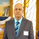Interesting in understanding the following formula, for which values of s and i dont understand. prev(s,i) =>
y=abs(round(i))
s Thanks.
ตอบกลับ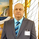what kind of statement the following codes are? A switch statement: #prev(s,i) =>
# y=abs(round(i))
# s

#calc_ttf( periods ) =>
# bp = highest( high, periods ) - prev( lowest( low, periods ), - periods )
# sp = prev( highest( high, periods ), - periods ) - lowest( low, periods )
# 100 * (bp - sp) / ( 0.5*( bp + sp) )

Thanks.
ตอบกลับ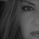You should be advanced into a Mod position:) You would be the only one that earned it in a hard way. Great and appreciate it work.
ตอบกลับsublimares2
thanks sublimares2. :)
ตอบกลับ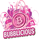Yet another useful indicator... Thanks LB
ตอบกลับ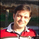LazyBear
Hey LB, is it possible for you to write a script that automatically identify's Gartley and Harmonic Patterns? Something like this script for MT4 http://www.fx-mt4.info/view-review/zup-v135-harmonic-patterns-indicator . It automatically draws on the chart the pattern as it's forming based on price and Fib levels until the pattern is complete.
ตอบกลับpcmourao
Pine script currently has no support for many of the constructs needed to do a script like this. Will post one as soon as we get the support (or I find a workaround).
ตอบกลับ